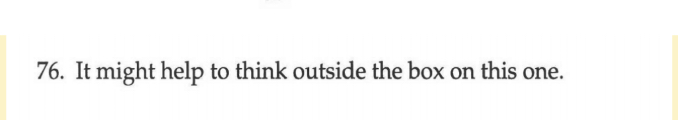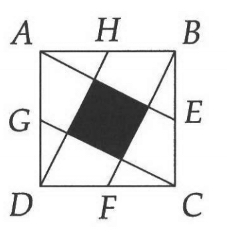# Justin Does Math

Right, this is correct.

Do you see the mistake you were making earlier? You originally had the opposite intuition, and thought maybe the rectangle length was longer than half the circumference.

Yeah. I think I was thinking about something like the curved line from Figure 1 as the length, and then was ALSO worried about the “angle” between the sectors causing a problem. So maybe I thought that like, maybe the baseline was that the rectangle length should be equal to half the circumference, and then there was this other factor that would make it greater than half. It was a pretty confused thought process.

You mentioned being concerned about the sectors being more spread out initially:

The part that is more spread out are the triangles though (the area part), NOT the line that makes up the circumference. Like, if they were spread out so that the circumference line was broken/no longer touching between the different triangles, then the length would end up being longer than the circumference. But the outer edge of the circle doesn’t end up being spread out in the rectangle - only the inner parts of the circle do.

1 Like

Tricky problem (final one in chapter). I’ll say more in a follow up postBTW I think you’re not supposed to use pythagorean theorem for this cuz that’s introduced in next chapter.

Since E, F, G, and H are midpoints of the sides, and since the shape is a square, I figured out that each short segment on the perimeter (line AH, line AB, line BE, and so on, around the square) is length 10.

My first attack of the problem went something like: well the area of the whole square is 20 x 20 = 400. Each short segment is length 10. So then I was like, well, the area of triangle ABE is \frac{1}{2}(10 * 20) = 100 and same with triangle CDG. So I was gonna subtract those from the area of the square. But then I ran into the issue that there are two quadrilaterals (to the top left and bottom right of the shaded area) that I haven’t accounted for, so that doesn’t work.

My second attack was to try to creatively overcounting and working my way back. What I mean is, there are big triangles “around” the shaded square that we can calculate the area of = namely ABE, BCF, CDG, and DAH. The area of those is 100. But if you just add them all up, you get 400, which is the area of the whole square. The issue is that there are four smaller triangle segments that are getting double counted. Now if you could figure out the area of those, you could compensate for the overcounting and thus figure out the area of the shaded area. I was unable to come up with a way of doing that without pythagorean theorem though.

I didn’t “get” there hint, don’t know what they have in mind.

I initially took their hint as meaning to extend lines outside the square. That’s a common technique. I quickly got a solution by looking at it that way:

Maybe you can figure out a solution from this pic.

But I don’t think this is the intended solution. I think the hint meant to try to calculate the area outside the black box – the white area.

I’m not sure if you’re asking for help or hints or not.

I solved it before reading your thoughts, but I used Pythagorean theorem the first time, since that seemed most intuitive to me. After reading that they didn’t intend Pythagorean to be used in the solution, I figured out a different way to solve it without using Pythagorean. I’m not sure if you want hints or help or anything though, so I don’t want to give more details.

Oh my bad. Hints/suggestions/help welcome. I guess start with hints. I looked at Elliot’s suggestion but haven’t figured out how to connect it to solving the problem.

Ok.

My non-pythagorean solution used similar triangles & ratios.

Did you figure out any new facts about the problem from looking at my pic?

List the tools/resources. Then worry about what you can do with them second.

I did not. I’m probably missing something obvious.

My hint. I blurred it in case someone doesn’t want to see it.

Start with your first attack. Then draw a line from E to GC, perpendicular to GC, and another from G to AE, perpendicular to AE.

OK so the basic technique here is to look at shapes that use some but not all the lines. You did that already when you saw the ABE triangle. To see that triangle, you ignored the HD and BF lines. Similarly, you might notice a triangle with A, B and the point touching the black square near E. To see that medium sized triangle you ignore the HD line. There’s also the small triangle. And if you look at my big pic, you can find some larger triangles.

Make sense so far?

Yeah

ok so do any of the larger triangles look useful? part of the way to see if they’re useful is by looking at how they can be broken up internally, so you can try to say the big shape equals several smaller shapes.

for example, if you find a large triangle that is the same area as the original square, you might be able to look at the components in it and know they add up to 400 area. that could give you a different set of components, besides the ones in the original square, that add to 400. so that’d help you figure it out.

and can you see any other shapes besides triangles?

I tried Anne’s hint. It is different from what I did, but I was able to find the area that way too (though I am not sure if the way that I did it was the way she intended).

Edit: actually, thinking about it, I am not sure if I solved it using Anne’s hint alone, or if I was taking advantage of things that I already knew from solving it myself and/or looking at Elliot’s image.

I can see that there’s a triangle here for example:

It would have the same area as original square (cuz the left side is 20 and the bottom is 40, so (20 * 40)/2 = 400). I’m getting stuck re: what to actually do with that info though, how to use it.

What are the shapes within this big triangle? And what are the shapes within the original square? Are they different? If they are different that’s useful.

To clarify what I mean, the original square contains:

1 black square
4 small triangles
4 trapezoids

my big triangle looks to have the same set of shapes, so not useful, i guess. i’ll try to find a different one

Hypothetically, suppose the big triangle contained 1 black square and 8 small triangles. Then you could figure some stuff out as follows:

Let S = black square, T = small triangle, and P = trapezoid

400 = 1S + 4T + 4P = 1S + 8T

And from that you’d be able to derive that T=P.

That’s just an example. T and P aren’t actually the same size.

Do you understand this?